# SPC/E model of water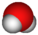This article is a 'stub' about water and/or ice. It has no, or next to no, content. It is here at the moment to help form part of the structure of SklogWiki. If you add material to this article, remove the {{Stub-water}} template from this page.

The SPC/E (extended simple point charge model)   is a slight reparameterisation of the SPC model of water, with a modified value for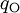$q_{\mathrm{O}}$. The molecule is modelled as a rigid isosceles triangle, having charges situated on each of the three atoms. Apart from Coulombic interactions, the molecules interact via long-range Lennard-Jones sites, situated on the oxygen atoms. The parameters are as follows:

 parameter value$\sigma$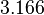$3.166$ Å$\epsilon$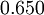$0.650$ kJ mol-1$r_\mathrm{OH}$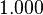$1.000$ Å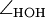$\angle_\mathrm{HOH}$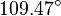$109.47^{\circ}$$q_{\mathrm{O}}$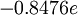$-0.8476 e$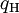$q_{\mathrm{H}}$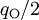$q_{\mathrm{O}}/2$ (charge neutrality)

The SPC/E model has a dipole moment of 2.351 D. (Ref. 1 Table I).

## Surface tension

The surface tension has been studied for the SPC/E model by Vega and Miguel 

## Phase diagram

### Plastic crystal phases

Recent simulations have demonstrated the existence of plastic crystal phases for the SPC/E model. 

## Shear viscosity

The shear viscosity for the SPC/E model is 0.729 mPa.s at 298 K and 1 bar  (experimental value 0.896 mPa.s ).